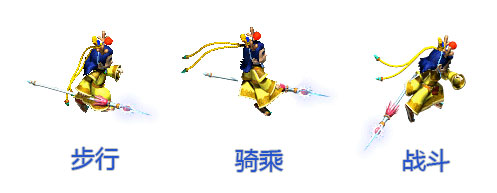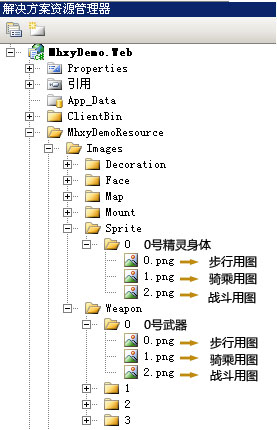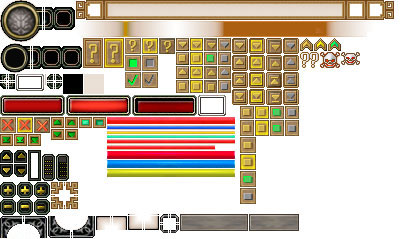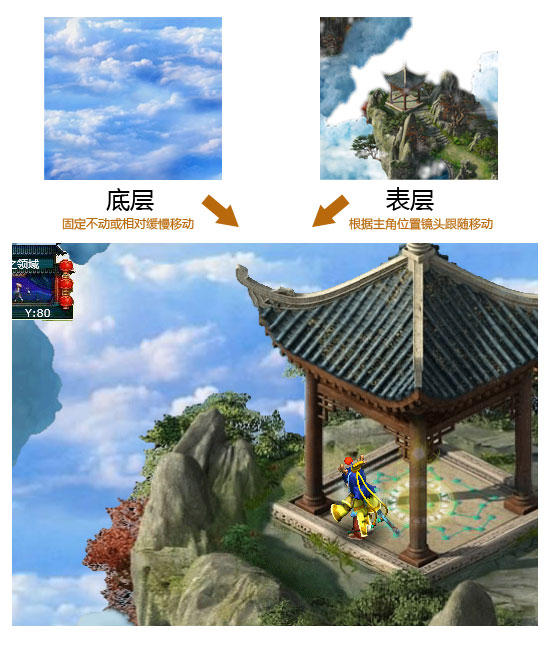底层为云彩，包含于Window窗口容器中，可固定不动或朝主角相反的方向1/10左右速度相对移动；表层则是正常的地图背景，主位式镜头跟随主角相对移动。通过此方式对场景进行分层处理可以实现华丽的2.8D效果，玩家在游戏中将亲历置身于天空之城般神奇的体验。而若在此基础上，将双背景换成双场景，那么《梦幻诸仙》的飞空系统不同样可以轻易实现？嘿嘿，又一次的印证了基于场景的游戏架构定能让游戏开发更加简单。

Point2D _sectionCenter;

/// <summary>

/// 获取或设置地图切片中心

/// </summary>

public Point2D sectionCenter {

get { return _sectionCenter; }

set {

if (!_sectionCenter.Equals(value)) {

ChangeMapSection(_sectionCenter = value);

}

}

}

/// <summary>

/// 更新呈现的地图切片

/// </summary>

int startSectionX, startSectionY, endSectionX, endSectionY;

//根据主角当前位置来加载周边地图切片

startSectionX = 0; endSectionX = 2;

} else if (leaderSection.X == SectionXNum - 1) {

} else {

}

startSectionY = 0; endSectionY = 2;

} else if (leaderSection.Y == SectionYNum - 1) {

} else {

}

int index = 0;

//取回已经超出主角周围9块范围的切片(x,y初始-1,第一次加载9)

tempSection[] tempSectionList = sectionList.Where(c => c.x < startSectionX || c.x > endSectionX || c.y < startSectionY || c.y > endSectionY).ToArray();

for (int x = startSectionX; x <= endSectionX; x++) {

for (int y = startSectionY; y <= endSectionY; y++) {

//9点位中假如已经有该点位的切片在则检查下一个

if (sectionList.Where(c => c.x == x && c.y == y).Count() > 0) {

continue;

} else {

//将超出范围的切片填充到新的位置上,并重置它们的x,y点位坐标值

tempSectionList[index].x = x;

tempSectionList[index].y = y;

//下载实际图片

Args = string.Format("{0},{1},{2}", index, x, y)

};

mapSection[tempSectionList[index].sectionNo].Source = GetProjectImage(string.Format("Images/MiniMap/{0}/Surface/{1}_{2}{3}", this.Code, x, y, FileType(MapFormat)));

};

int tempIndex = Convert.ToInt32(args), tempX = Convert.ToInt32(args), tempY = Convert.ToInt32(args);

if (tempSectionList[tempIndex].x == tempX && tempSectionList[tempIndex].y == tempY) {

mapSection[tempSectionList[tempIndex].sectionNo].Source = GetWebImage(string.Format("Images/Map/{0}/Surface/{1}_{2}{3}", this.Code, tempX, tempY, FileType(MapFormat)));

}

};

Canvas.SetLeft(mapSection[tempSectionList[index].sectionNo], x * SectionWidth);

Canvas.SetTop(mapSection[tempSectionList[index].sectionNo], y * SectionHeight);

index++;

}

}

}

}

<!--传送点-->

<Teleports>

<Teleport ID="0" Code="0" X="2820" Y="2040" Z="184" ToScene="0" ToX="105" ToY="49" ToDirection="2" Tip="传送到:比武场"/>

<Teleport ID="1" Code="0" X="2455" Y="1680" Z="164" ToScene="0" ToX="57" ToY="95" ToDirection="6" Tip="传送到:武门殿"/>

<Teleport ID="2" Code="0" X="4270" Y="1530" Z="156" ToScene="0" ToX="99" ToY="83" ToDirection="2" Tip="传送到:空中亭阁"/>

<Teleport ID="3" Code="0" X="835" Y="1510" Z="154" ToScene="0" ToX="88" ToY="79" ToDirection="0" Tip="传送到:空中亭阁"/>

</Teleports>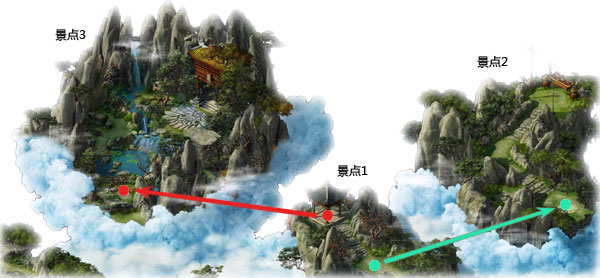再复杂的游戏设计也离不开最普通的游戏架构基础，光有强大的场景仍然无法支撑游戏的完美表述；因此，下一节我会在此Demo的基础上进一步丰富游戏，赋予游戏更多的生命与灵气。赶快加入到Silverlight游戏开发的行列中来吧，世界将因你而变得更精彩！posted on 2010-04-14 14:36  深蓝色右手  阅读(8189)  评论(15编辑  收藏